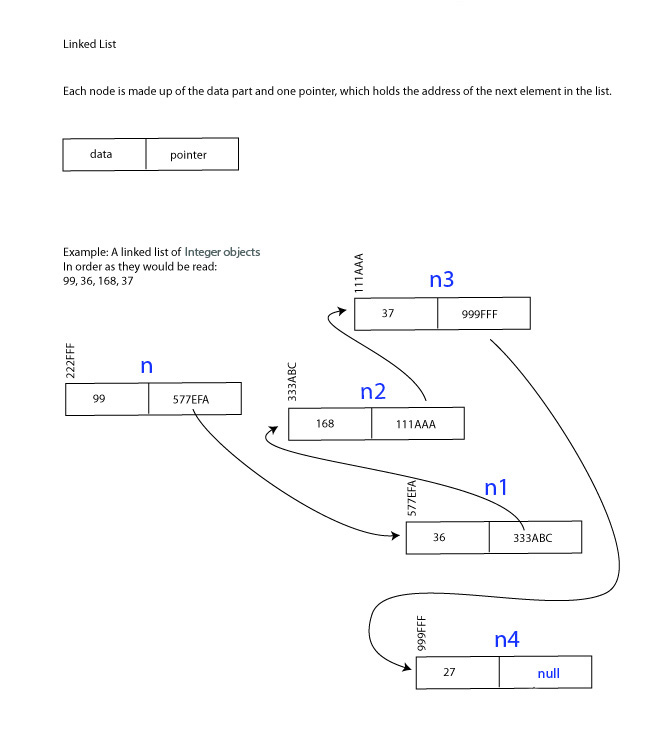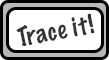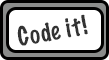Logout

(5.1.11) (5.1.12)

D.4.6

Construct algorithms that use reference mechanisms.

Teaching Note:

Sample Question:

sdfsdfsf

JSR Notes:

This assessment statement immediately jumps right into quite sophisticated code, but taken one step at a time, it all makes sense. The other thing to note at this stage is the difference between this, and D.4.10, and D.4.11, and D.4.12, the other three "algorithm" D HL Extension assessment statements.

Context of this in terms of algorithms responsibility (Repeated later on):

D.4.6 - Here, we aren't necessarily thinking about a list, we just want to see generally how linking is achieved through self-referential pointers. (Though what we look at here is obviously most ultimately usable in either trees or lists.)

D.4.10 - The employment of self-referential classes for implementations of general lists - and not necessarily thinking stacks or queues, but certainly these are useful ways of using lists.

D.4.11 - The use of self-referential classes in lists via the specific ADTs ArrayList and LinkedList. And though able to treat lists as stacks and queues, also searching, deleting, adding in order through whole lists.

And D.4.12 is for tracing through such algorithms.

### Basic Referential Algorithms

At a basic level, this is how Nodes (of a linked list or binary tree) can be made, to link and point to one another.

Node n = new Node();
Node n1 = New Node();
Node n2 = new Node();
Node n3 = New Node();
//etc.

//Link the nodes one to another as a list, using the setNext( )s.
n.setNext(n1);
n1.setNext(n2);
n2.setNext(n3);
//etc.
//or in the case of a tree
n.setLeft(n1);
n.setRight(n2);
//etc.

//Traverse through the linked nodes from one node to another (as a list), using getNext( )s.
Node secondStep = n.getNext( );
Node thirdStep = secondStep.getNext( );
Node fourthStep = thirdSetp.getNext( );
//etc.

***It is the setNext( ) and getNext() methods that take in as a parameter, or return another Node, which are key. The secret is that Nodes are object, and objects are references, so if the "next" attribute of a Node is another Node, then, in fact what "next" is is the *address* of the next Node.***### Actual Referential Mechanism Algorithms

Even though applied usage of specific list classes (like in D.4.11) is what is most focused on the Topic D Extension, this particular assessment statement is pretty clear with what it wants: "Construct algorithms that use reference mechanisms" i.e. general algorithms.

So one way or the other, general or specific, here we go, with Java self referential algorithms.

Taking one step at a time, helped along by a good white board diagram, you'll see that each is quite logical. Learning, and even memorizing these will help you understand referential mechanisms and lists, and also help you with all related construction and tracing questions from this point forward.

A Typical Node Class

```1public class Node {
2    private Object obj = null;  //The "data" part. By being Object, all objects will work with this class.
3    private Node next = null;     //The key part of this class for linking; the self-referential "next" node in the list.
4
5    public Node(){
7    }
8
9    public Node(Object obj, Node node){
10        this.obj = obj;
11        this.next = node;
12    }
13
14    public void setNext(Node node) {     //To set what the next node will be.
15        this.next = node;
16    }
17
18    public void setData(Object obj) {
19        this.obj = obj;
20    }
21
22    public Node getNext() {     //To allow accessing the next Node in the list.
23        return next;
24    }
25
26    public Object getObj() {
27        return obj;
28    }
29}
30```

A Basic List Class

```1public class List {
1
2    private Node head = null;   //So essentially this is all a List is; just a single "head" node.
3
4    public List(){
5
6    }
7
10    }
11
17            node.setNext(head);   //Stick the new node onto the front by pointing to the present head (even if head is null).
18            head = node;          //But then need to make it the new head.
19        }
20    }
21
22
24        if(isEmpty()){
26        }
27        Node current = head;               //Need to traverse to the end of the list.
28        while(current.getNext() != null){  //We know we are not there yet as long as current does not point to null.
29            current = current.getNext();
30        }
31        current.setNext(node);     //Once to the end, set the pointer of that node to the new one.
32    }
33
34
35    public Node getAndRemoveFromFront(){
36        if(isEmpty()){
37            return null;
38        }
39
40        Node temp = head;          //Will need to keep track of the head before it is lopped off.
42        return temp;        //Now we do what we said we would and return the (now former) head.
43    }
44
45
46    public Node getAndRemoveFromEnd(){
47        if(isEmpty()){
48            return null;
49        }
50        Node current = head;      //Need to traverse to end, starting at head, but...
51        Node previous = null;     //Will also need to keep track of "previous" for linking purposes later.
52        while(current.getNext() != null){   //As with other traversing, keep moving up as long as you can.
53            previous = current;             //Each step along the way, previous can be current current.
54            current = current.getNext();    //Then the current becomes the next.
55        }
56        previous.setNext(null);   //Once at the end, break the link to the last node (current) with this line.
57        return current;           //Return this, which is now no longer accessible from the list.
58    }
59
60    public boolean isEmpty(){     //Link to D.4.10 - check for empty list
61        return head == null;     //Needed to be checked above each method in case the list is empty.
62    }
63} ```
A Simple Testing Class
```1public class MainForTestingList {
2
3    public static void main(String[] args) {
4        List list = new List(null);
8        System.out.println(list.getAndRemoveFromEnd().toString());
9        //should print out Bob
10      System.out.println(list.getAndRemoveFromEnd().toString());
11      //should print out Sam
12    }
13}
```---------------------------------

Maybe a bit more here, but connected to further Topic D assessment statements.

`2195   deja vu  Basics of Lists and Trees`
```2135 code Linked Lists - Generic Node Class & List Class